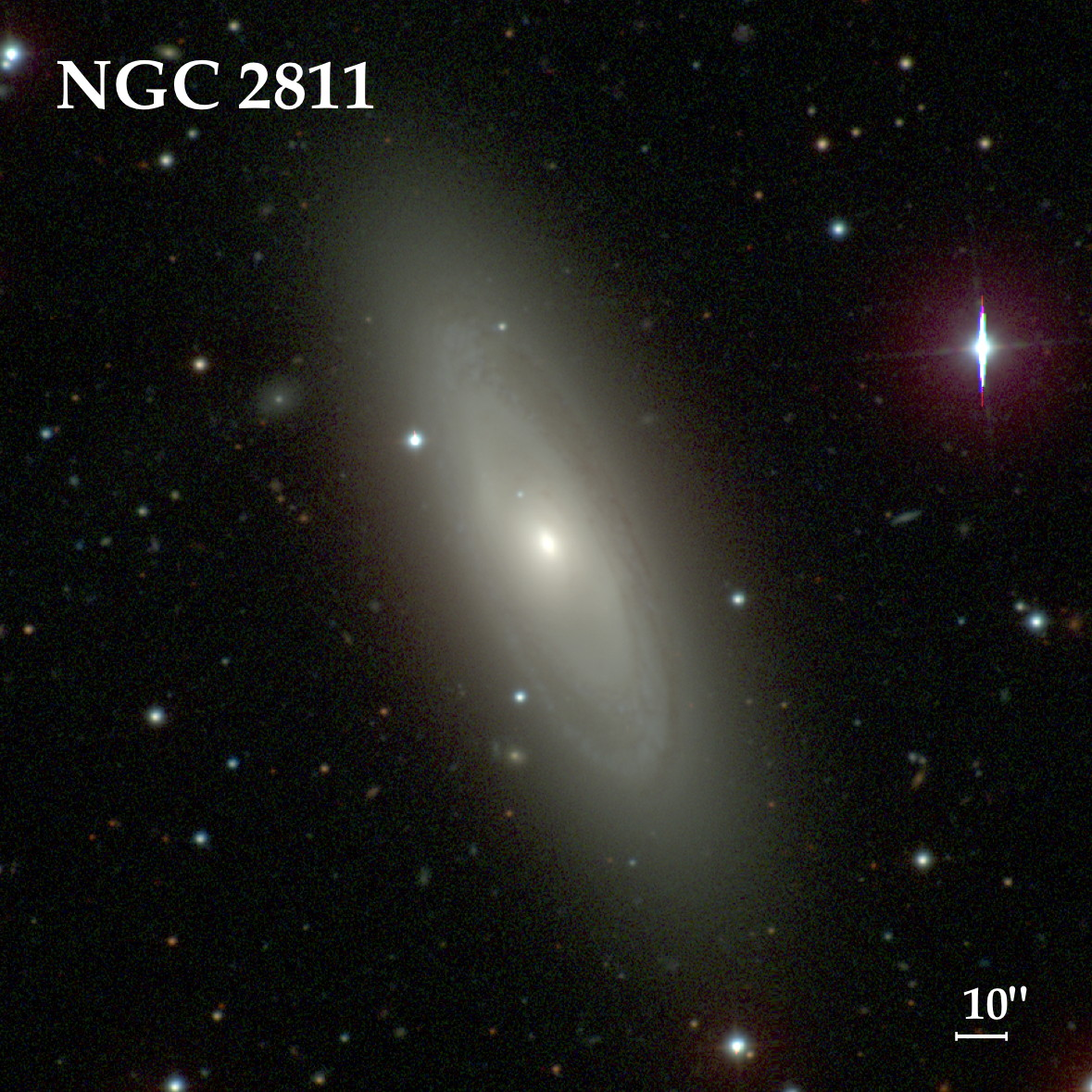The Carnegie-Irvine Galaxy Survey (CGS)Data Products

Images

1. original FITS images (B, V, R, I)

2. color-composite image (original)

3. color-composite image (star-cleaned)

1. surface brightness (B, V, R, I)

2. ellipticity (B, V, R, I)

3. position angle ( B, V, R, I)

4. A4 harmonic amplitude (B, V, R, I)

5. B4 harmonic amplitude (B, V, R, I)

6. m = 1 relative amplitude (B, V, R, I)

7. m = 1 phase angle (B, V, R, I)

8. m = 2 relative amplitude (B, V, R, I)

9. m = 2 phase angle (B, V, R, I)

10. color profile (B-I, V-I, R-I)